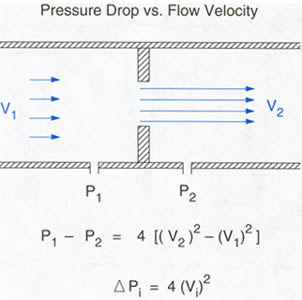Figure 46f Schematic illustrating the relation between flow velocity and pressure drop across a discrete obstruction. Proximal to the obstruction, flow is laminar (parallel arrows) and velocity V1 is normal. At the level of the obstruction, and just beyond, flow remains laminar and velocity V2 is increased. the pressure drop P1-P2 across the stenosis can be calculated from the velocities by the modified Bernoulli equation. Since V1 usually is considerably smaller than V2, the instantaneous pressure drop can be determined from the instantaneous velocity(V) in the stenotic jet.
click to close window